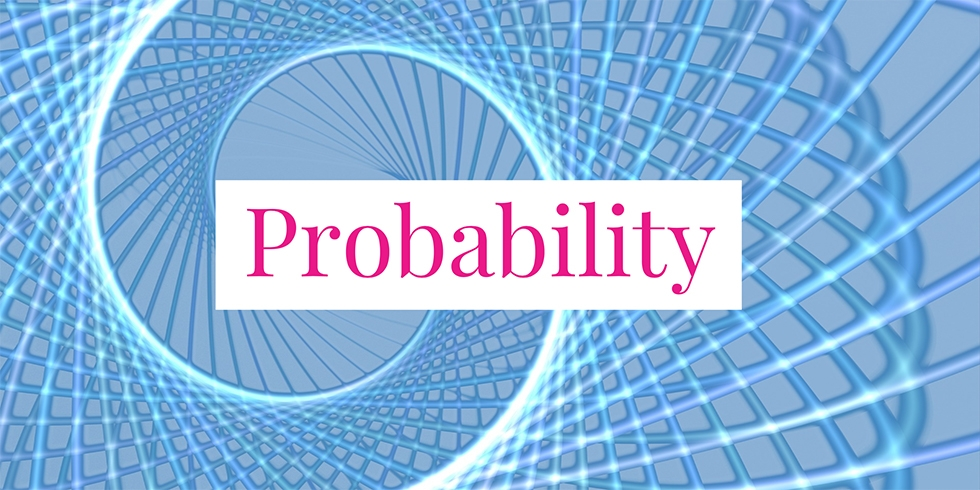# Independent Events of Playing Cards## Problem 732

A card is chosen randomly from a deck of the standard 52 playing cards.

Let $E$ be the event that the selected card is a king and let $F$ be the event that it is a heart.

Prove or disprove that the events $E$ and $F$ are independent.Add to solve later

### Definition of Independence

Events $E$ and $F$ are said to be independent if
$P(E \cap F) = P(E) \cdot P(F).$

Intuitively, this means that an occurrence of one does not change the probability that the other occurs. Mathematically, this can be seen as follows.

If $P(E) \neq 0$, then independency implies
$P(F \mid E) = \frac{P(F \cap E)}{P(E)} = \frac{P(F)P(E)}{P(E)} = P(F).$

## Solution.

We prove that the events $E$ and $F$ are independent.
First, we have $P(E \cap F) = 1/52$ because there is only one King of hearts card in the deck of 52 cards.

Since there are four kings, we have $P(E) = 4/52$. As there are $13$ heart cards, we have $P(F) = 13/52$.
Thus, we see that
$P(E)P(F) = \frac{4}{52} \cdot \frac{13}{52} = \frac{1}{52} = P(E \cap F).$ This implies that the events $E$ and $F$ are independent.Add to solve later

### More from my site

#### You may also like...

This site uses Akismet to reduce spam. Learn how your comment data is processed.

###### More in Probability##### Jewelry Company Quality Test Failure Probability

A jewelry company requires for its products to pass three tests before they are sold at stores. For gold rings,...

Close